# Spearman coefficient of rank correlation

Jump to: navigation, search

A measure of the dependence of two random variablesand, based on the rankings of the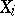's and's in independent pairs of observations. Ifis the rank ofcorresponding to that pair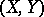for which the rank ofis equal to, then the Spearman coefficient of rank correlation is defined by the formulaor, equivalently, by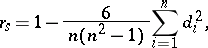where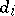is the difference between the ranks ofand. The value oflies between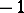and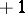;when the rank sequences completely coincide, i.e.,; and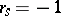when the rank sequences are completely opposite, i.e.,. This coefficient, like any other rank statistic, is applied to test the hypothesis of independence of two variables. If the variables are independent, then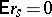, and. Thus, the amount of deviation offrom zero gives information about the dependence or independence of the variables. To construct the corresponding test one computes the distribution of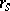for independent variablesand. When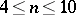one can use tables of the exact distribution (see , ), and whenone can take advantage, for example, of the fact that asthe random variableis asymptotically distributed as a standard normal distribution. In the latter case the hypothesis of independence is rejected if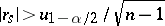, where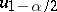is the root of the equationand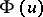is the standard normal distribution function.

Under the assumption thatandhave a joint normal distribution with (ordinary) correlation coefficient,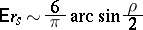as, and therefore the variable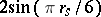can be used as an estimator for.

The Spearman coefficient of rank correlation was named in honour of the psychologist C. Spearman (1904), who used it in research on psychology in place of the ordinary correlation coefficient. The tests based on the Spearman coefficient of rank correlation and on the Kendall coefficient of rank correlation are asymptotically equivalent (when, the corresponding rank statistics coincide).